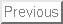Next:Bibliography, Table and Figures

A study of dissipative, shoaling high-frequency internal waves in shallow water

James Lerczak, Clinton Winant and Myrl Hendershott

Scripps Institution of Oceanography, CCS 0209
University of California at San Diego
La Jolla, California 92093-0209
http://www-ccs.ucsd.edu/iwaves/

The evolution of shoreward propagating, high-frequency, mode one internal waves was studied in a nearshore region where water depths ranged from 30 to 15 m. The data for this study were obtained from upward-looking acoustic doppler current profilers (ADCPs) deployed off of Mission Beach, California in 1996 and 1997 (Table 1). The ADCPs were rigidly mounted on the bottom, allowing vertical velocities to be unambiguously distinguished from the more energetic horizontal velocities. The bathymetry was approximately planar, with an onshore bottom slope,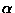, of approximately 0.01. Data were analyzed over the period 26 September to 23 October (26.2 days) in 1996 and 4 September to 18 October (44.0 days) in 1997.

Ten frequency bands, having equal widths on a logarithmic scale, between 0.0167 and 0.3 cycles per minute (T = 60 to 3.33 min) were studied. Within this range, coherences between vertical velocity, w, and horizontal velocities,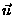, were high. This range contained approximately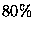of the mid-depth w signal variance. The ADCP signal to noise variance ratio was approximately 20, where the noise level was estimated to be the high-frequency noise floor of w. Vertical velocities were studied because, when measured from a stable platform, they are a very sensitive index of high-frequency internal wave variability (Marsden et al., 1994), and are less likely to be contaminated by other dynamics, such as barotropic eddies, than horizontal velocities. While w of long-wavelength waves is much weaker than, the maxima ofof mode one waves occur near the surface and near the bottom. These regions of the water column were not well-covered by the ADCPs, which sampled the center 67 to 75% of the water column. In contrast, the single maximum of w of mode one waves occurs near the thermocline. This maximum was always detected by the ADCPs.

To isolate the mode one internal wave variability, the vertical velocities at each mooring were decomposed into complex empirical orthogonal functions (cEOFs) (Wallace and Dickinson, 1972) within each of the ten frequency bands for consecutive blocks of data 212 minutes (2.84 days) in duration. Some of the results of the cEOF analysis are briefly summarized here. From 70 to 90% of the variance in each band was explained by the first cEOF mode,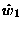, indicating the high frequency wavefield was dominated by mode one waves. The vertical structure ofwas consistent with mode one waves, having a single, mid-column maximum. The average depth of this maximum varied approximately linearly from 7 to 12.5 m as water depth changed from 15 to 30 m. The relative phases betweenand horizontal velocities in the upper and lower water column were consistent with onshore propagation (leading w by 90o near the surface, and lagging w by 90o near the bottom). The square of the ratio of major to minor axis horizontal velocities coherent with(ellipticity) was as high as 16, and major axis angles were nearly cross-isobath, consistent with uni-directional, cross-isobath propagating waves.

The variance of the amplitude ofas a function of frequency and water depth was calculated. For linear waves, this variance is an estimate of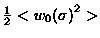, where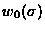is the vertical velocity amplitude of the mode one internal wave at the depth of maximum variance within the frequency band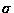. Since cEOFs were estimated for consecutive ensembles of length 2.84 days, a smoothed time series of the internal wave variance, summed over the frequency range, was obtained (Fig. 1). The time series were quite coherent from mooring to mooring, and the variance clearly decreased with decreasing water depth. The frequency spectra of cEOF amplitude at each mooring had a peak at approximately 0.11 cpm (T = 9 min) (Fig. 2). The amplitude of this peak decreased with decreasing water depth, however, there was no obvious frequency shift in the peak or change in the spectral shape. The variance, summed over the frequency range, and averaged over ensembles, decreased nearly monotonically with decreasing water depth (Fig. 3).

This decrease is well described by a simple shoaling model with linear dissipation in which, for time independent propagation of waves up a beach with constant slope,, the divergence of the energy flux is balanced by dissipation. Thus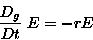(1)

in which E is the depth-integrated, time-averaged energy density, and the substantial derivative is taken in a frame moving at the waves group velocity,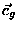, so that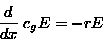(2)

For a fluid with constant stratification, the amplitude,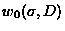, of the wave consequently varies with water depth according to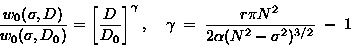(3)

where N is the buoyancy frequency of the fluid. For inviscid progagation (r = 0), the amplitude is inversely proportional to water depth. The variance would then increase by a factor of 4, as a wave propagates from a water depth of 30 m to 15 m. This was clearly not observed in our data. When linear dissipation is included in the model, the amplitude may increase or decrease as the wave shoals, depending on the size of r. We estimate r by minimizing the mean square error between the observed variance at each depth, and the estimated variance using the above shoaling model, the spectrum at 30 m (Fig. 2), and an assumed buoyancy frequency of 0.263 cpm obtained from the estimated long-wave phase speed at different water depths (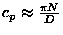). The resulting estimates for r were 2.0 x 10-4 and 1.6 x 10-4s-1for 1996 and 1997, respectively. These values correspond to decay time scales of 1.4 and 1.8 hours, respectively, which are comparable to the typical time (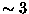hours) it takes a wave packet to pass from the 30 m to the 15 m moorings (cross-shore distance = 1.5 km). Such time scales are consonant with those use by Pringle and Brink, in prep., in somewhat deeper water depths.Next:Bibliography, Table and Figures

1998-09-08